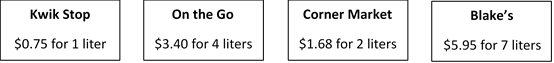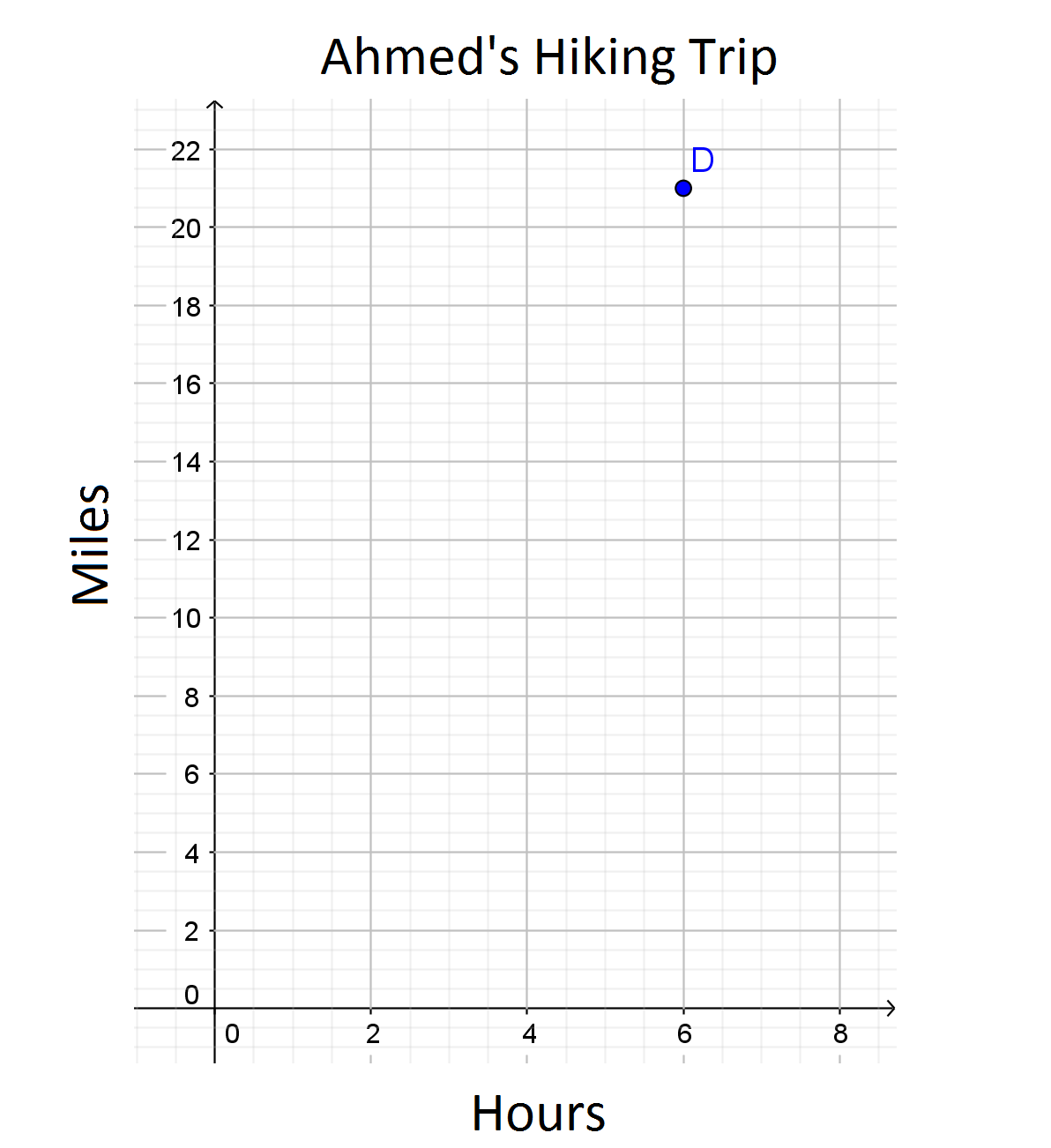# Proportional Relationships

## Objective

Determine if relationships are proportional or non-proportional.

## Common Core Standards

### Core Standards

?

• 7.RP.A.2.A — Decide whether two quantities are in a proportional relationship, e.g., by testing for equivalent ratios in a table or graphing on a coordinate plane and observing whether the graph is a straight line through the origin.

?

• 6.RP.A.3

## Criteria for Success

?

1. Determine if two quantities are in a proportional relationship by creating and analyzing tables, graphs, and equations of the relationship.
2. Justify, with tables, equations, graphs, or explanations, why two quantities are or are not in a proportional relationship.
3. Understand that quantities with the same unit rate are in a proportional relationship (MP.3).
4. Understand that perimeter and side length of regular polygons are in a proportional relationship.

## Tips for Teachers

?

This Lesson extends on Lesson 8 by having students make the determination if a relationship is proportional or non-proportional, and to justify their reasoning.

#### Fishtank Plus

• Problem Set
• Student Handout Editor
• Vocabulary Package

## Anchor Problems

?

### Problem 1

Is the perimeter of a square proportional to the side length of a square?
Is the area of a square proportional to the side length of a square?

### Problem 2

At Sunny’s Market, soda water costs \$2.55 for 3 liters.

Which stores below sell soda water at the same price per liter as Sunny’s Market?### Problem 3

Ahmed hiked at a constant speed for 6 hours and covered 21 miles. This is represented in the coordinate plane below.Which coordinate points, when graphed on the plane above, would be in a proportional relationship to Ahmed’s hike? Select all that apply.

a.  (0, 3.5)

b.  (1, 3.5)

c.  (2, 7)

d.  (3, 14)

e.  (4, 15)

f.  (5, 17.5)

g.  (8, 28)

## Problem Set

?The following resources include problems and activities aligned to the objective of the lesson that can be used to create your own problem set.